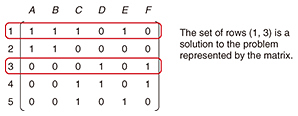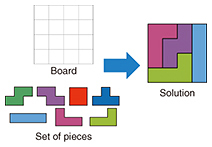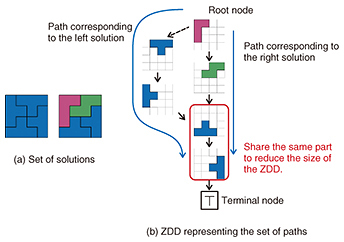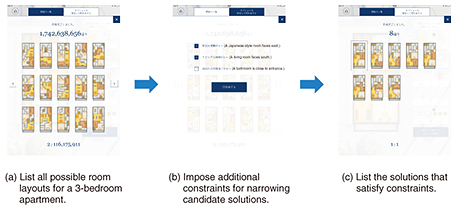##Feature Articles: Communication Science that Enables corevo®—Artificial Intelligence that Gets Closer to People

# Efficient Algorithm for Enumerating All Solutions to an Exact Cover Problem

## Abstract

We introduce an algorithm that finds all solutions to an exact cover problem. Many real-world tasks including designing apartment layouts and electric circuits can be formulated and solved as exact cover problems. Our algorithm can solve exact cover problems up to 10,000 times faster than the previous method. Moreover, our method compresses and stores all solutions and so can efficiently find the solutions that satisfy several constraints. Therefore, our algorithm can efficiently find good solutions to exact cover problems found in the real world.

Keywords: algorithm, data structure, exact cover problem

## 1. Exact cover problems

Most people have had some experience with puzzles such as crosswords, Sudoku, and Rubik’s cubes. Many computer science researchers have tried for decades to design algorithms* that can solve these puzzles by using computers. As a result, several efficient algorithms have been developed that can solve these puzzles much faster than humans can.

However, puzzles that are difficult for humans are also difficult for computers. Rubik’s cube and Sudoku are known to be NP-complete problems, and they become drastically more difficult as the problem size increases. For example, if we increase the number of squares on each face of a Rubik’s cube to 16, 25, 36,…, then solving the puzzle requires exponentially more time. This inherent difficulty of puzzles is why researchers are continuing to pursue more efficient algorithms that can solve large and complex puzzles.

We have developed a new algorithm that can efficiently find all the solutions of an exact cover problem, a fundamental problem in combinatorics. An example of an exact cover problem is to find a set of rows of a binary matrix X (a matrix whose elements are either 0 or 1), where the selected rows must contain exactly one numeral 1 in every column. An example of an exact cover problem is shown in Fig. 1. If the matrix shown in the figure is given as the input, then the set of rows (1, 3) has exactly one 1 in every column and is thus a solution to the exact cover problem. An exact cover problem may have multiple solutions. This example problem also has another solution (2, 3, 5). Our algorithm can find all of the solutions to this exact cover problem.Fig. 1. An exact cover problem.

Our algorithm can also be used to find all the solutions to puzzles that can be formulated as exact cover problems. A polyomino is a puzzle that involves arranging tiles on a board using a set of pieces consisting of square cells, where all pieces are used and each cell on the board is covered by square cells, with no spaces left uncovered and no pieces overlapping. This is a typical example of a puzzle that can be formulated and solved as an exact cover problem.

The example in Fig. 2 shows how tetromino puzzles, a kind of polyomino where every piece is made by connecting four square cells, can be formulated as an exact cover problem. Every column of the problem matrix corresponds to a cell of the 4×4 input board (thus, there are 16 columns), and every row corresponds to an arrangement of a piece. If the cell corresponding to the j-th column is covered by the arrangement of a piece corresponding to the i-th row, we set matrix element xij to 1. Otherwise, we set xij to 0. A solution to the exact cover problem formulated in this way represents a set of arrangements of pieces covering all of the cells on the board with no overlapping pieces. Hence, it is a solution to the tetromino puzzle.Fig. 2. Example of polyomino (tetromino) puzzle.

Other than puzzles, several real-world problems can be formulated and solved as exact cover problems. For example, designing the layout of an apartment can be regarded as solving a polyomino puzzle, where every piece corresponds to a room. The problem of designing the layout of an electric circuit can also be formulated and solved as an exact cover problem that is similar to a polyomino puzzle. Hence, our algorithm can find good solutions to these problems.

 * Algorithm: A computation procedure for solving a problem. A computer can solve various problems by running algorithms.

## 2. Algorithm for solving exact cover problems

Exact cover problems are known to be NP-complete. Donald E. Knuth’s algorithm DLX is accepted as a state-of-the-art algorithm for finding all solutions to an exact cover problem . DLX solves a problem by performing an exhaustive search. Given input binary matrix X, DLX selects the first row and checks whether or not it is a solution to the exact cover problem. If it is not a solution, then it selects the pair of the first and second rows and checks whether or not that combination is a solution. DLX repeatedly adds rows to the current set of rows and checks whether it is a solution. If adding the i-th row to the current set makes more than two 1’s appear in a column, then it cannot be a solution. Therefore, DLX removes the most recently added row from the set and adds a different row to the set to continue the search procedure. In this way, algorithm DLX finds all solutions by repeatedly adding and deleting rows to the set of rows to check all possible combinations of rows. Although the exhaustive search procedure is straightforward, DLX accelerates the search by exploiting a specialized data structure.

DLX can efficiently find all solutions to an exact cover problem if the number of solutions is limited. However, since it is an exhaustive search method, it takes an excessive amount of time if there are many solutions. Real-world exact cover problems sometimes have a huge number of solutions. For example, small polyomino puzzles can have more than one billion solutions.

Our new algorithm improves DLX to achieve practical speeds even when there are huge numbers of solutions . The key idea of the proposed algorithm is to store all the solutions found in a search. If an exact cover problem has many solutions, then it is highly likely that they will be similar in several ways. For example, there are many solutions to a polyomino puzzle that has the same placement of pieces on the left half of the input board. If we memorize all the solutions, they can be used as hints to find similar solutions and thus accelerate the search procedure.

Although storing all the solutions may increase the search speed, memorizing billions of solutions in a naïve manner is unrealistic. Our algorithm uses the data structure called a zero-suppressed binary decision diagram (ZDD) to store the set of found solutions. A ZDD represents the set of solutions as a directed graph. We show in Fig. 3(b) an example ZDD that represents the set of two solutions (Fig. 3(a)) of the tetromino puzzle in Fig. 2. This ZDD has two paths that start from the root node and end at terminal node T. These two paths correspond to the two solutions. Since a ZDD shares partial paths, it yields a small graph that can represent huge numbers of solutions. The ZDD in the figure shares two common edges of paths in order to reduce the size of the graph.Fig. 3. ZDD representing a set of solutions to an exact cover problem.

For example, a tetromino tiling problem with a board size of 10×10 has 7,213,560,548,906,621 solutions. If we use the naïve representation, the computer would require 100 petabytes (1018 bytes) of memory. In contrast, with our ZDD approach, the set is represented as a directed graph with 16,476,396 nodes. Such a ZDD requires only 300 megabytes (106 bytes) of memory and could therefore be handled by a modern smartphone. This compressed representation drastically speeds up the computation process. Our method is up to 10,000 times faster than DLX at finding all solutions to exact cover problems. Moreover, our algorithm is the first one capable of finding all the solutions to a tetromino tiling problem with a 12×12 board size. We confirmed that there are 13,664,822,582,333,502,156,627,512 solutions to the problem.

## 3. Application to real-world problems

Since our method represents the set of found solutions as a ZDD, we quickly identify solutions that satisfy several conditions. With this feature, our method can find good solutions to real-world problems. For example, the problem of designing the layout of an apartment is known to be an exact cover problem . By using our algorithm to construct a ZDD that represents the set of all possible floor plans, and then interactively adding conditions that match the buyer’s requirements, we can efficiently find satisfactory arrangements. A demonstration system that uses our algorithm to find acceptable apartment layouts is shown in Fig. 4. Because our algorithm can find all possible room arrangements for an apartment, we can browse them as a list. Moreover, we can add conditions on possible floor plans and efficiently identify floor plans that satisfy the additional conditions.Fig. 4. Demonstration system for designing layouts of an apartment.

## 4. Conclusion

Our new algorithm for solving exact cover problems is fast and can efficiently store all the solutions. These features enable the interactive discovery of desirable solutions by setting conditions in practical situations, which is especially beneficial for problems such as finding a desired floor plan. We are planning to improve the data structure in order to find the optimum solutions to practical exact cover problems.

## References

 D. E. Knuth, “Dancing Links,” Millennial Perspectives in Computer Science, pp. 189–214, 2000. M. Nishino, N. Yasuda, S. Minato, and M. Nagata, “Dancing with Decision Diagrams: A Combined Approach to Exact Cover,” Proc. of the 31st AAAI Conference on Artificial Intelligence, San Francisco, CA, USA, Feb. 2017. A. Takizawa, Y. Miyata, and N. Katoh, “Enumeration of Floor-plans Based on a Zero-suppressed Binary Decision Diagram,” International Journal of Architectural Computing, Vol. 13, No. 1, pp. 25–44, 2015.Masaaki Nishino Research Scientist, NTT Communication Science Laboratories. He received a B.E., M.E., and Ph.D. in informatics from Kyoto University in 2006, 2008, and 2014. He joined NTT in 2008. His current research interests include data structures, natural language processing, and combinatorial optimization. Norihito Yasuda Senior Researcher, NTT Communication Science Laboratories. He received a bachelor’s degree in integrated human studies and a master’s degree in human and environmental studies from Kyoto University in 1997, and 1999, and a D.Eng. in computational intelligence and system science from the Tokyo Institute of Technology in 2011. He joined NTT in 1999. He also worked as a research associate professor with the Graduate School of Information Science and Technology, Hokkaido University, in 2015. His current research interests include discrete algorithms and natural language processing. Shin-ichi Minato Professor, Graduate School of Information Science and Technology, Hokkaido University. He received a B.E., M.E., and D.E. in information science from Kyoto University in 1988, 1990, and 1995. He worked in the NTT laboratories from 1990 until 2004. He was a visiting scholar in the Computer Science Department at Stanford University, USA, in 1997. He joined Hokkaido University as an associate professor in 2004, and has been a professor since October 2010. He has also worked as a visiting professor at the National Institute of Informatics since 2015. His research interests include efficient representations and manipulation algorithms for large-scale discrete structures such as Boolean functions, sets of combinations, sequences, and permutations. He was a research director of the JST ERATO MINATO Discrete Structure Manipulation System Project from 2009 to 2016 and is now leading a Grant-in-Aid for Scientific Research (KAKENHI) project of the Japan Society for the Promotion of Science (JSPS) until 2020. He is a senior member of the Institute of Electronics, Information and Communication Engineers (IEICE) and the Information Processing Society of Japan (IPSJ), and a member of the Institute of Electrical and Electronics Engineers (IEEE) and the Japanese Society for Artificial Intelligence (JSAI). Masaaki Nagata Senior Distinguished Researcher, Group Leader, NTT Communication Science Laboratories. He received a B.E., M.E., and Ph.D. in information science from Kyoto University in 1985, 1987, and 1999. He joined NTT in 1987. His research interests include morphological analysis, named entity recognition, parsing, and machine translation. He is a member of IEICE, IPSJ, JSAI, the Association for Natural Language Processing, and the Association for Computational Linguistics.

↑ TOP